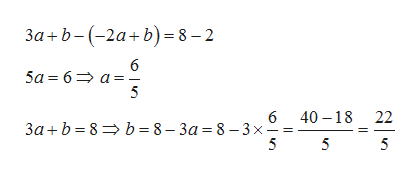# A linear function is such that ?(3) = 8 and ?(−2) = 2. Find the formula for the linear function.

Question

A linear function is such that ?(3) = 8 and ?(−2) = 2. Find the formula for the linear function.

check_circle

Step 1

Let the linear function be f(x)=ax+b

Since , we have f(3)=8 and f(-2)=2, so we have two equation in x

Step 2

On solving these equations , we get the values a and b.help_outlineImage Transcriptionclose3a+b--2a b) 8-2 6 5a 6 a- 5 6 b = 8- 3a = 8 -3x - 5 40 18 22 3ab 8 5 5 fullscreen
Step 3

On substituting the values of a and ...

### Want to see the full answer?

See Solution

#### Want to see this answer and more?

Solutions are written by subject experts who are available 24/7. Questions are typically answered within 1 hour.*

See Solution
*Response times may vary by subject and question.
Tagged in

### Equations and In-equations